# Introduction

The kirkegaard package contains a number of helper function for ggplot2. These are designed to save time, but do not genrally expand the capabilities of what a skilled ggplot2 user can do. As such, they do not constitute an extension. This documents gives some examples of the functions. All the functions begin with `GG_` so they are easy to find.

## GG_scatter

This is a convenience function to easily make scatterplots that add useful information such as the observed correlation in the plot and case names. To use it, give it a data.frame and the names of the two variables:

``GG_scatter(iris, "Petal.Length", "Sepal.Width")``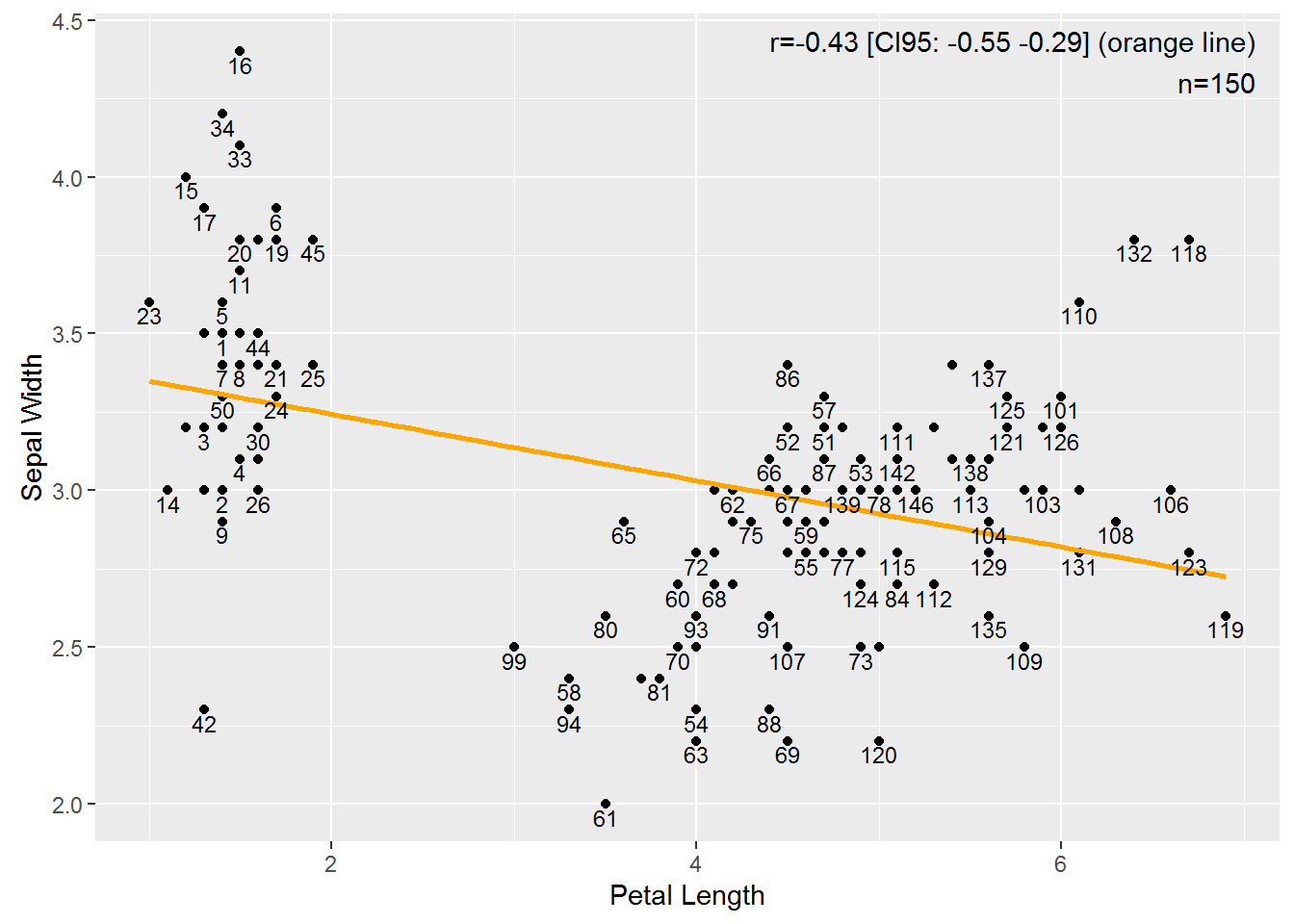By default, the rownames are used as case names. We can turn this off with `case_names = F`:

``GG_scatter(iris, "Petal.Length", "Sepal.Width", case_names = F)``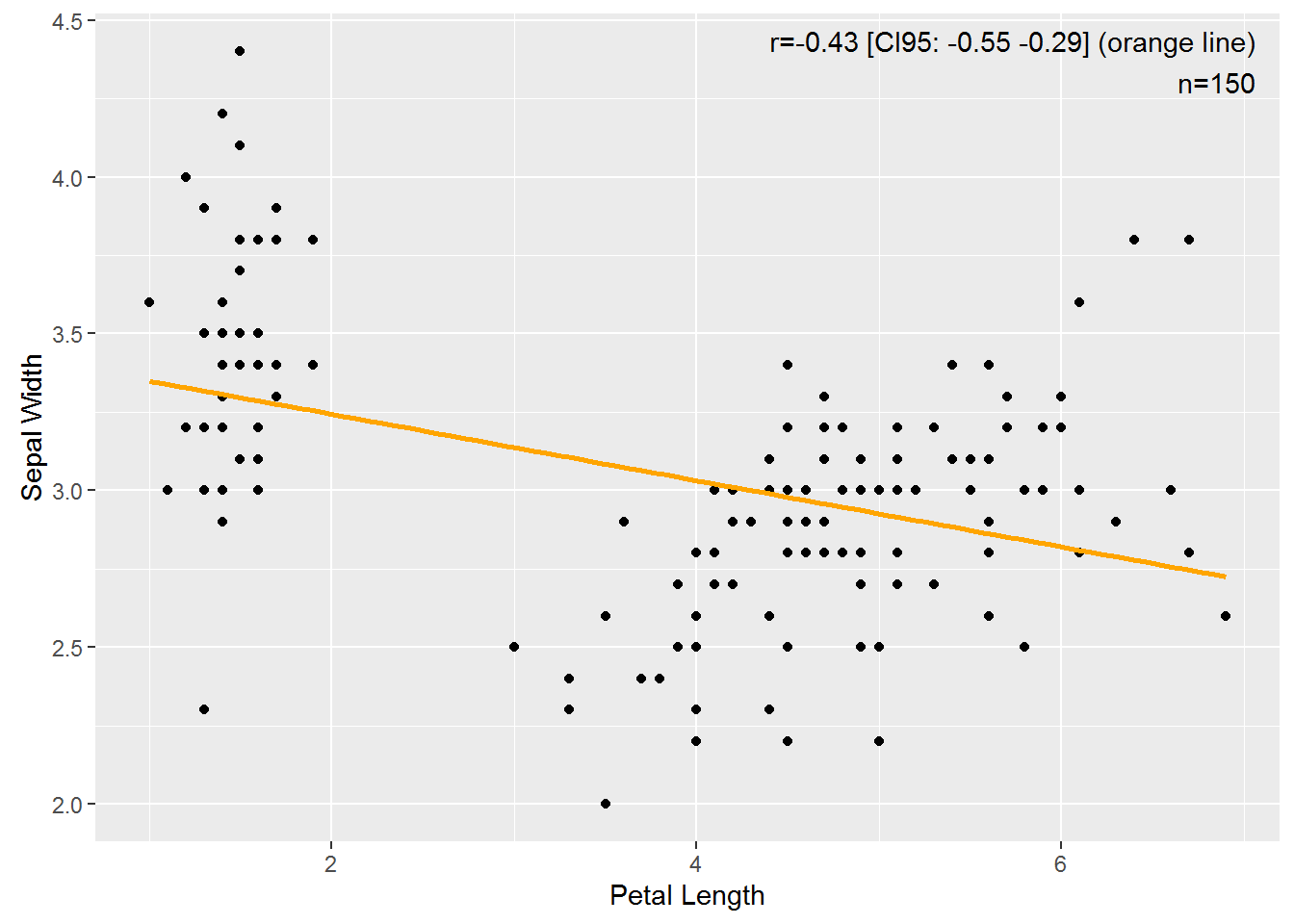The correlation, its confidence interval and the sample size is automatically shown in the corner where the data is least likely to be. One can control the position using `text_pos`:

``GG_scatter(iris, "Petal.Length", "Sepal.Width", case_names = F, text_pos = "bl")``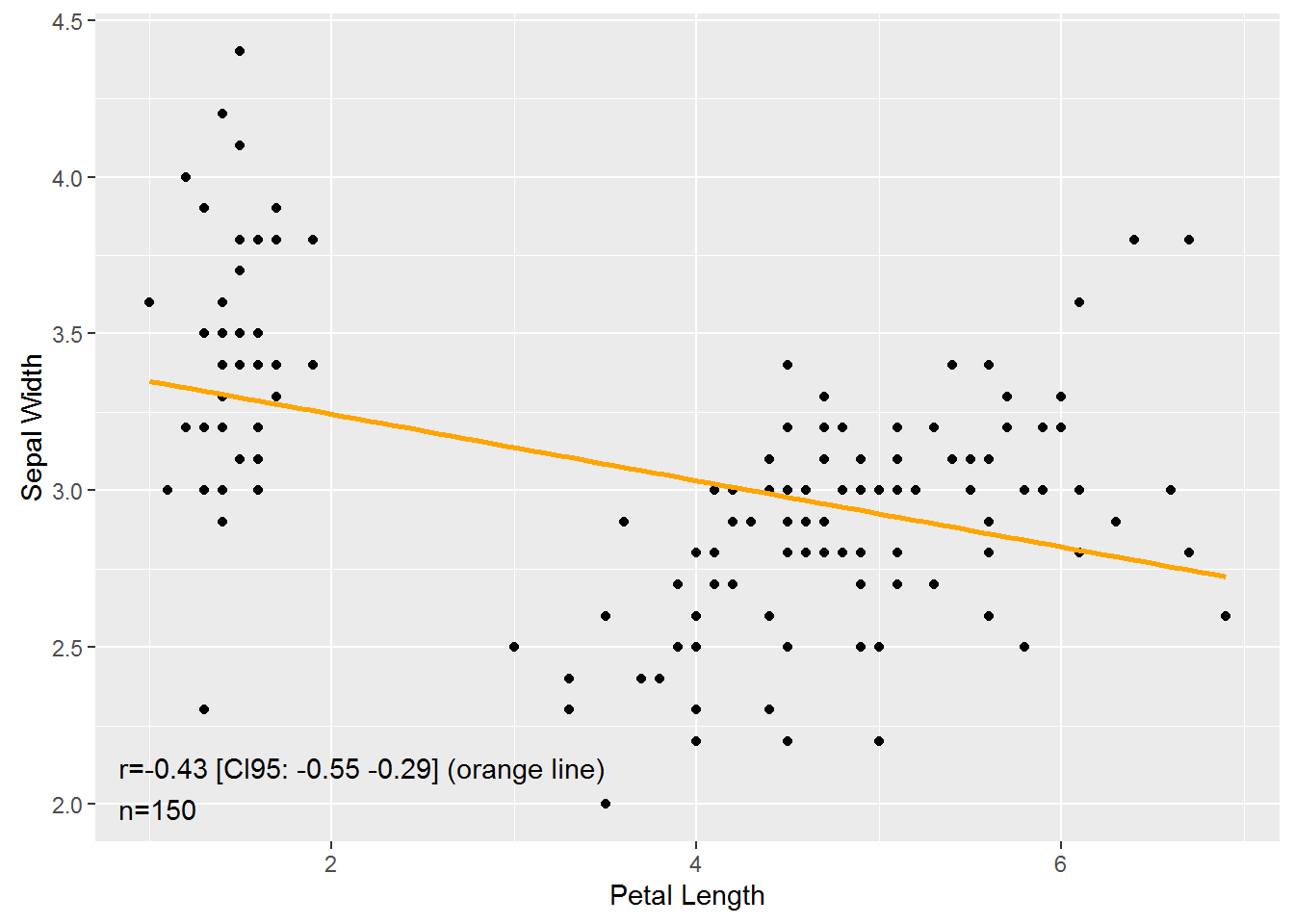One can add weights which are automatically mapped to the size of the points using `weights`:

``````set.seed(1)
GG_scatter(iris, "Petal.Length", "Sepal.Width", case_names = F, weights = runif(150))``````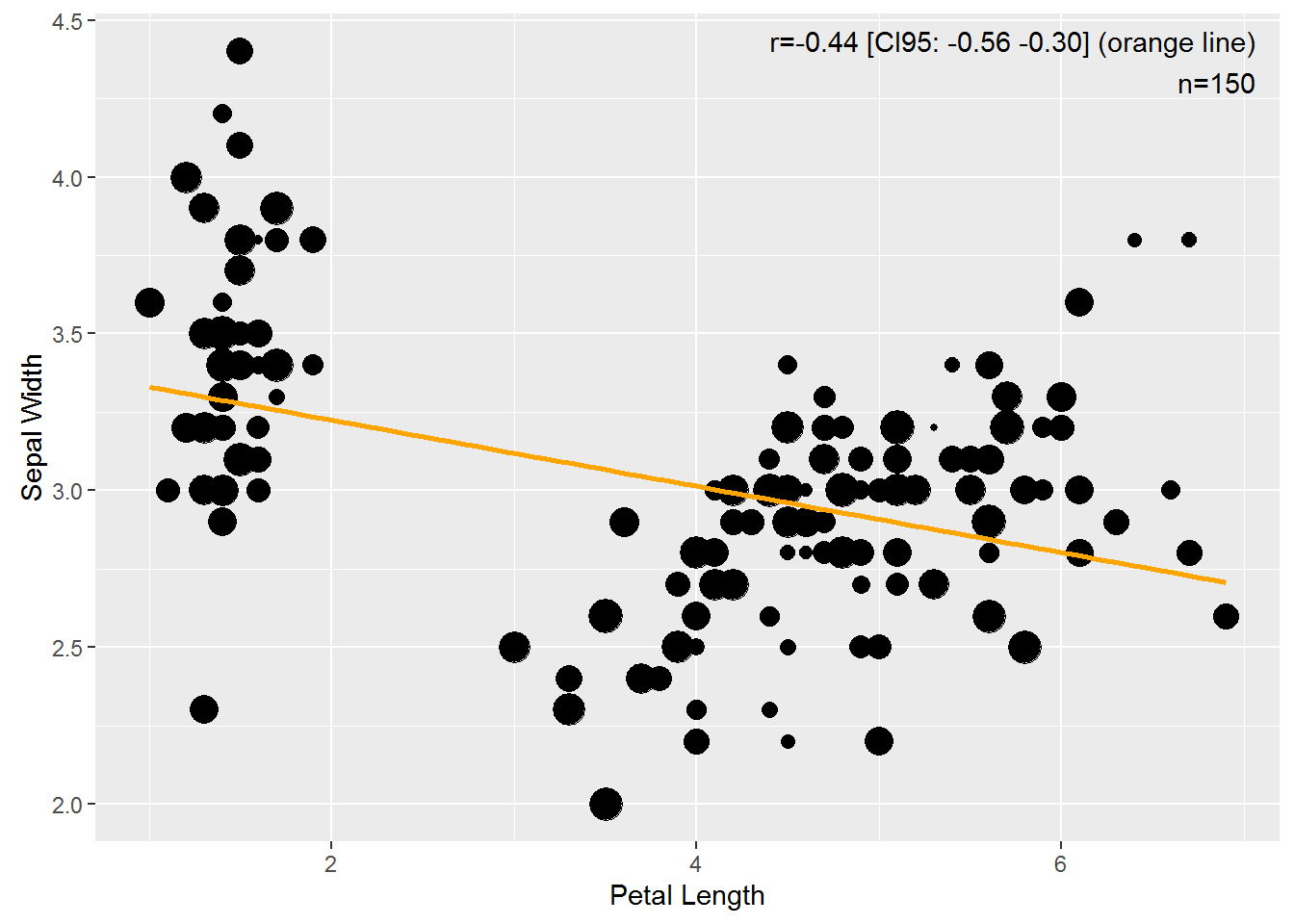Note that the correlation is a weighted correlation and automatically uses the supplied weights as well.

If we want to use other case names, they can be supplied using `case_names_vector`:

``````set.seed(1)
GG_scatter(iris, "Petal.Length", "Sepal.Width", case_names_vector = sample(letters, replace = T, size = 150))``````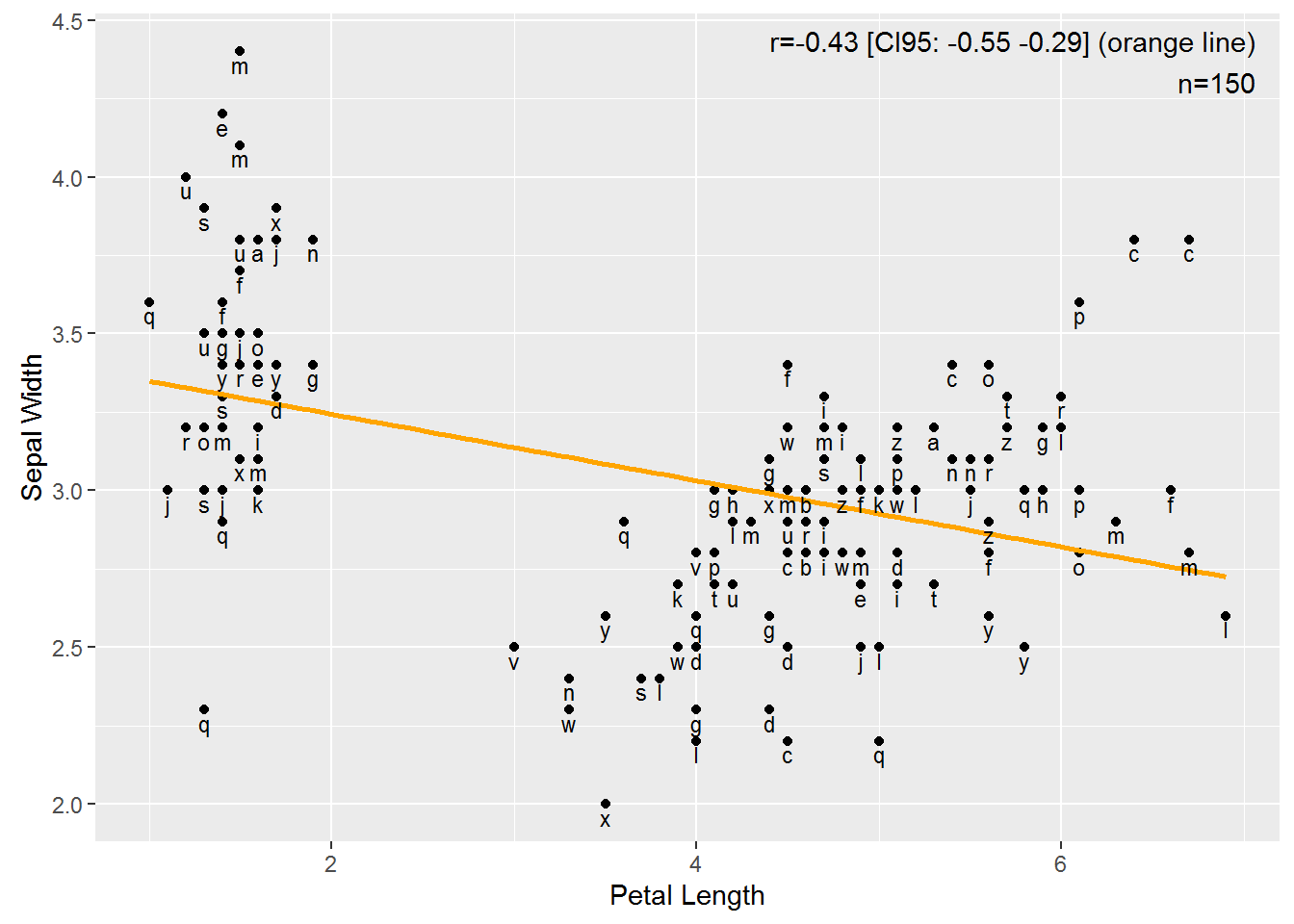We can use another confidence interval by passing it to `CI`:

``GG_scatter(iris, "Petal.Length", "Sepal.Width", case_names = F, CI = .99)``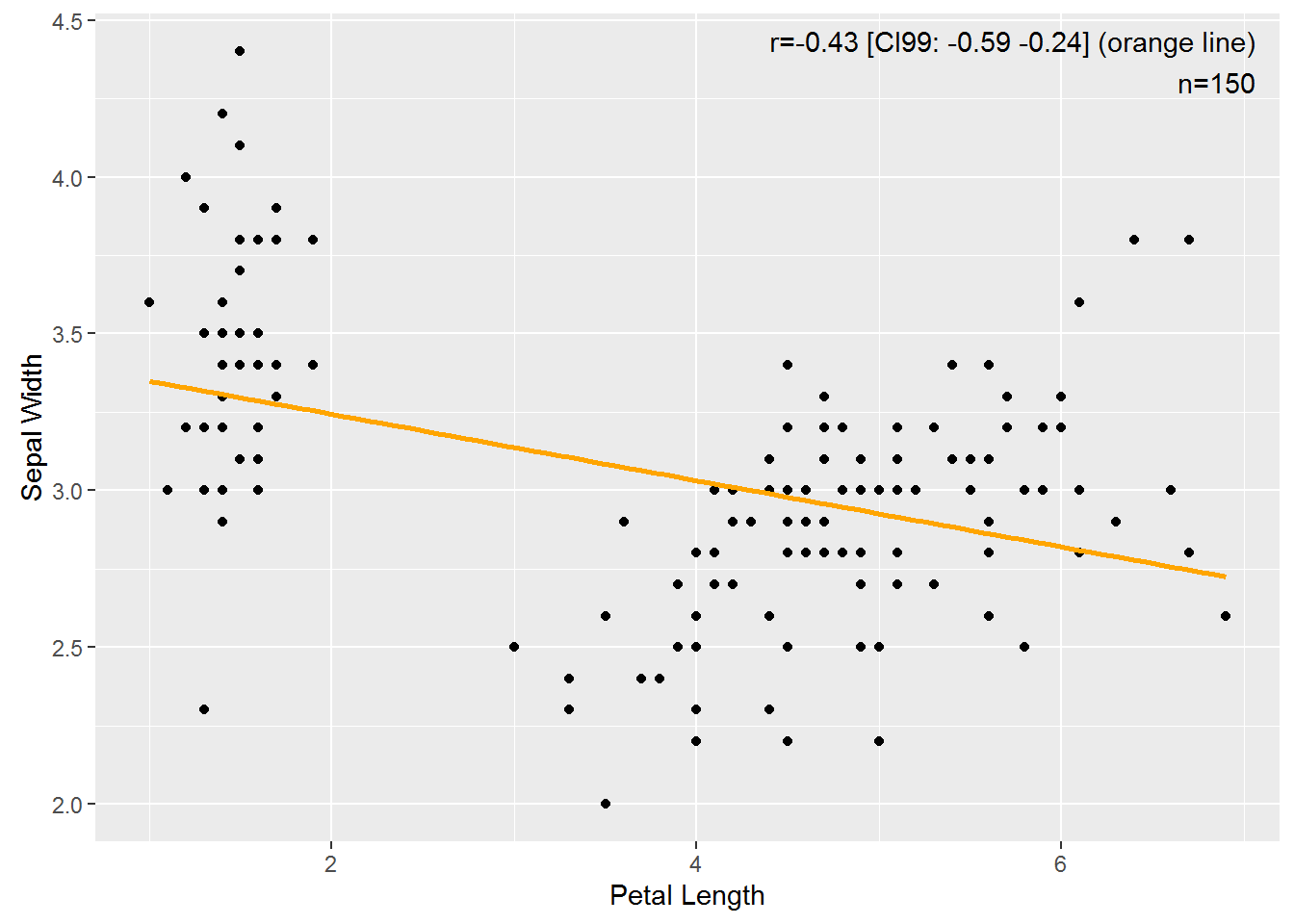## GG_denhist

It is frequently desired to make density or histograms of data distributions. `GG_denhist` makes both and combines them:

``GG_denhist(iris, "Sepal.Length")``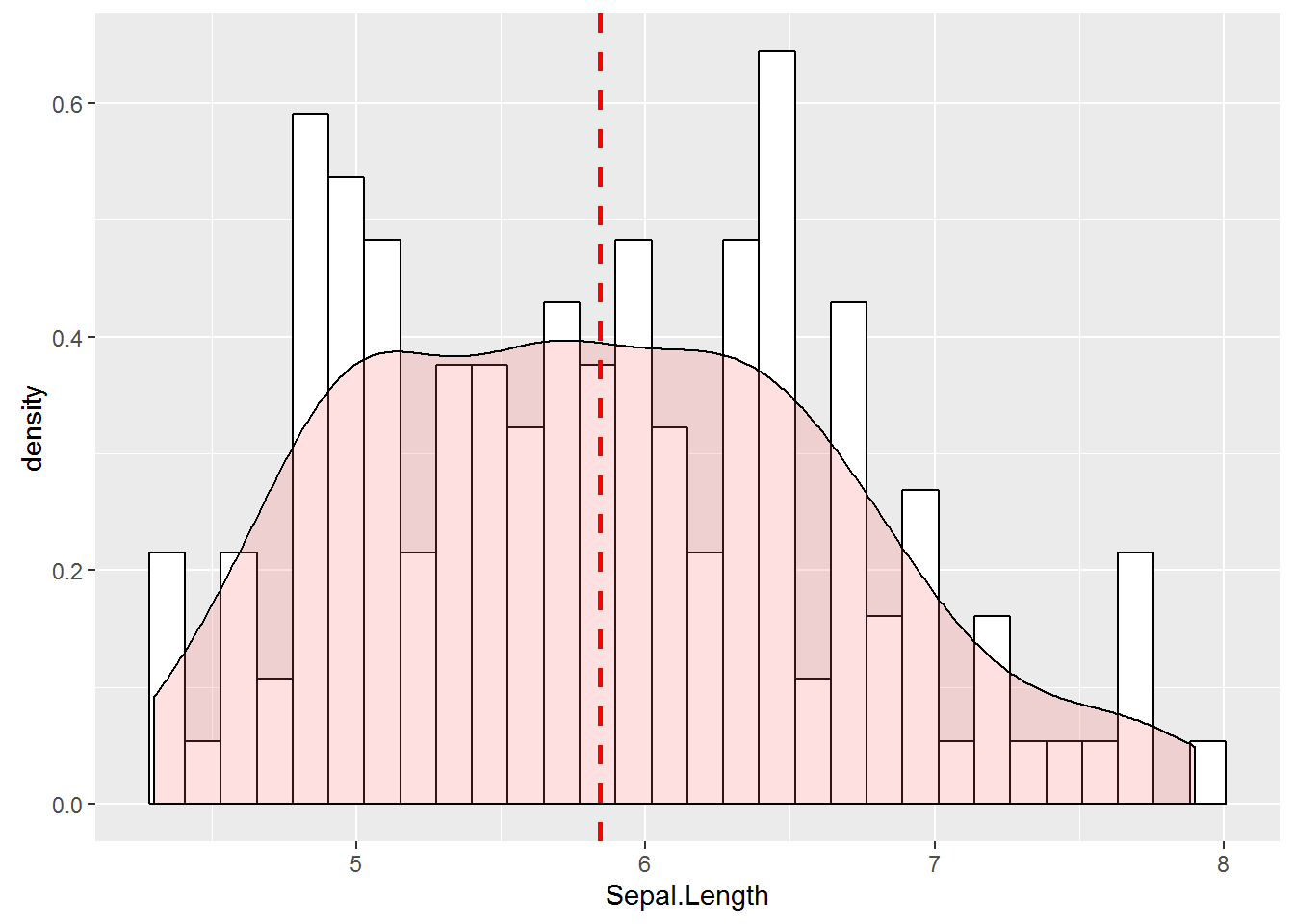Currently, the y scale is nonsensical and only the relative differences are meaningful. In the future, the y scale will be the proportion.

A vertical one is automatically plotted for the mean. We can supply another function to `vline` if we want another kind of average:

``GG_denhist(iris, "Sepal.Length", vline = median)``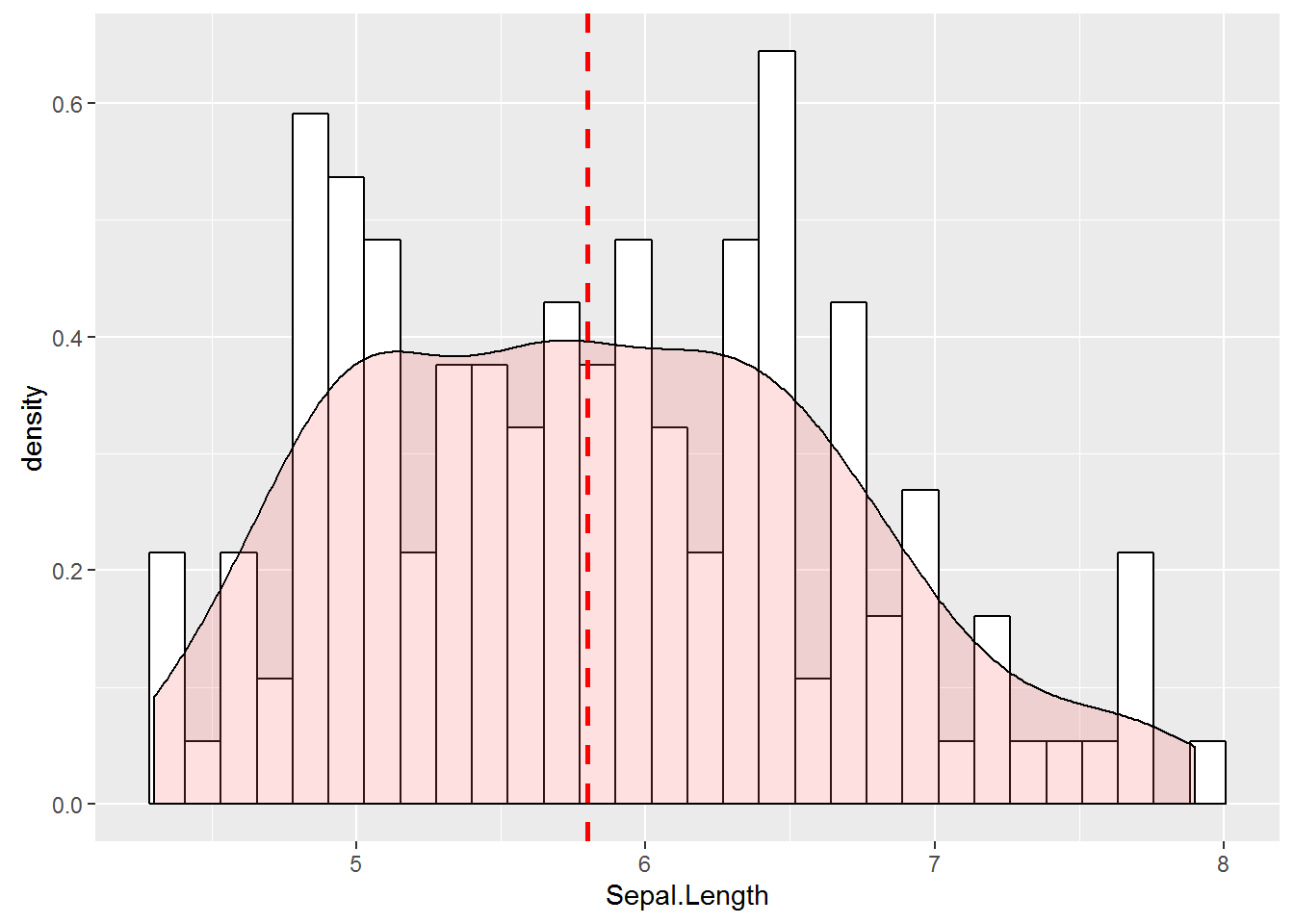We can supply a groping variable if we want to compare groups:

``GG_denhist(iris, "Sepal.Length", group = "Species")``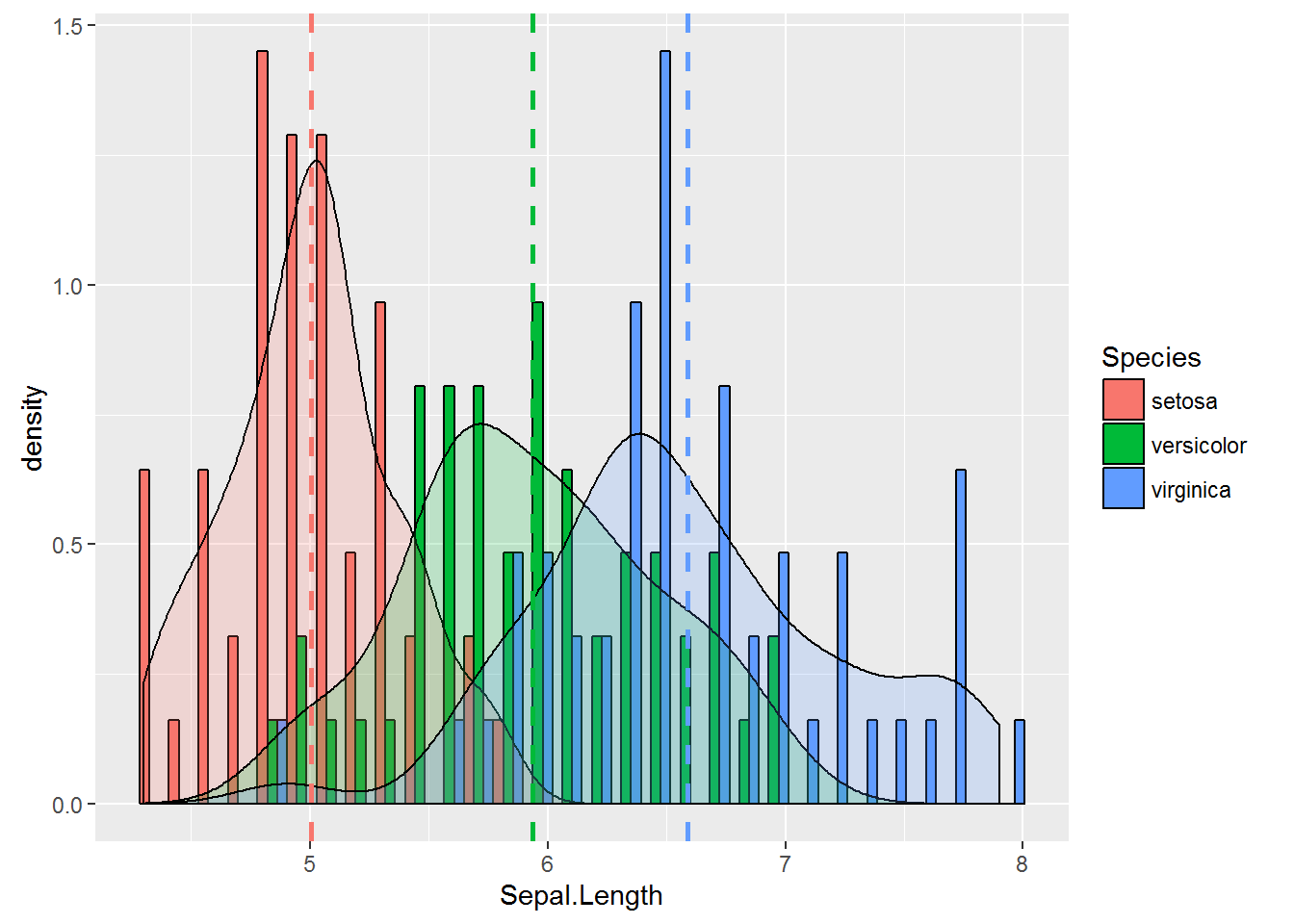## GG_group_means

Examining group averages is a frequent task. `GG_group_means` makes this easier, supply a data.frame, the name of the data variable and the name of the grouping variable:

``GG_group_means(iris, var = "Sepal.Length", groupvar = "Species")``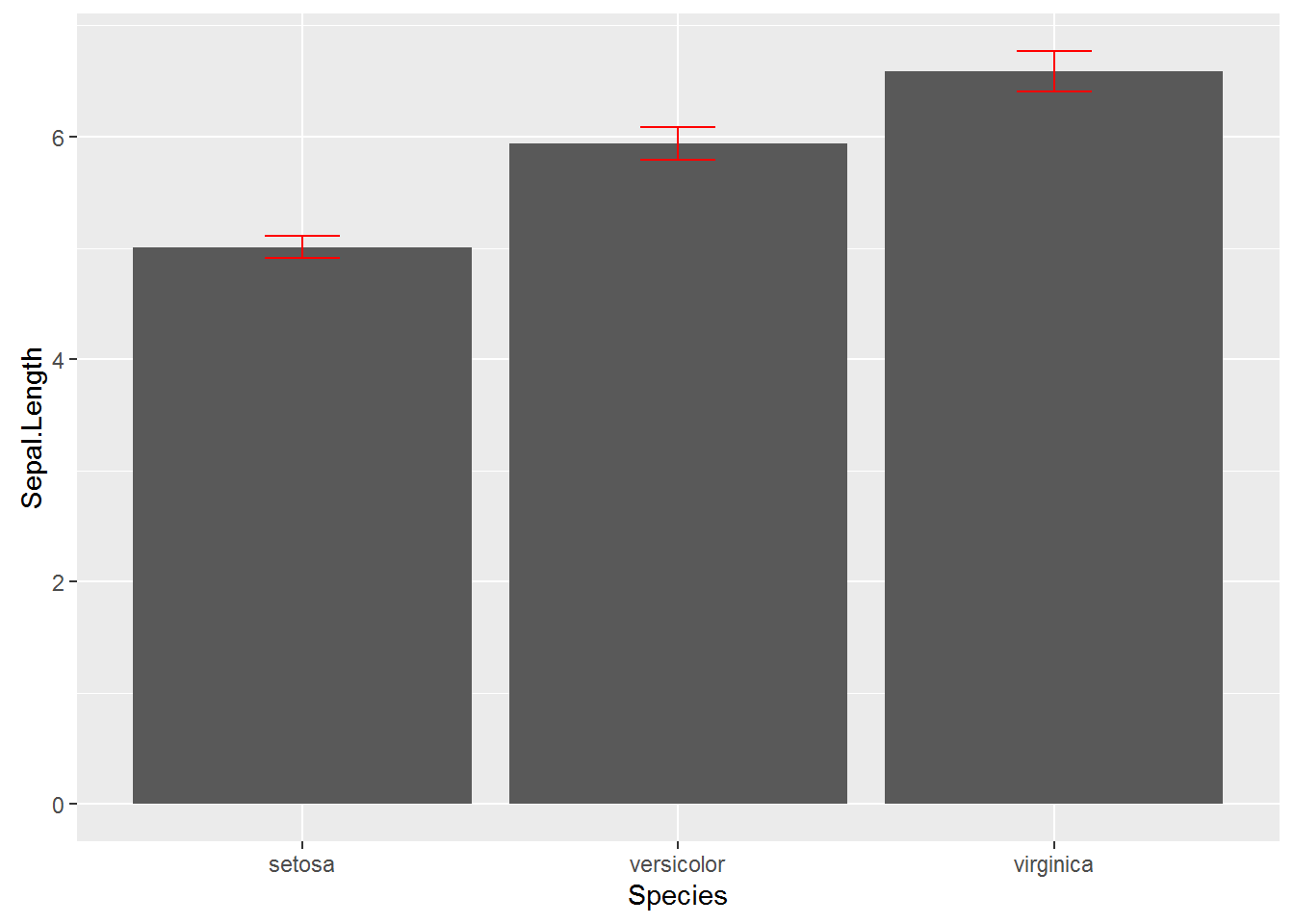There are a number of built in visualizations which can be controlled by `type`:

``````#bar (default)
GG_group_means(iris, var = "Sepal.Length", groupvar = "Species", type = "bar")````````````#point
GG_group_means(iris, var = "Sepal.Length", groupvar = "Species", type = "point")``````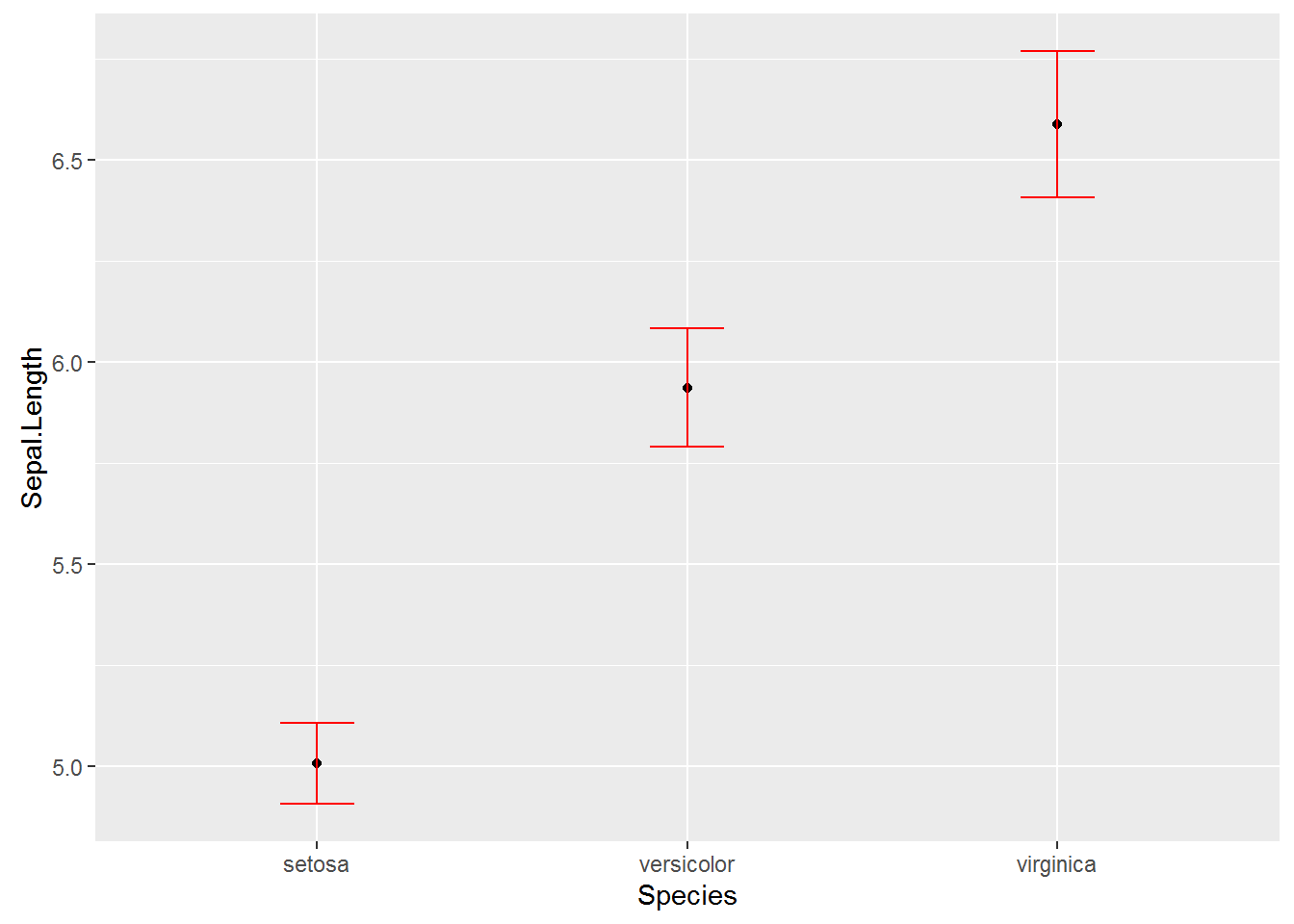``````#points
GG_group_means(iris, var = "Sepal.Length", groupvar = "Species", type = "points")``````